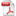# Implementing Multiplication And Division In Optics

Case ID:
UA18-191
Invention:

This invention is an all-optical approach to implementing mathematical operations on a circuit board in a computer. It uses the non-linear response to light of certain types of thin film materials in a novel approach to multiply & divide numbers in an all-optical mathematical circuit. The approach is an analogue method which implies a constant time for mathematical operation and will not increase as the problem size increases in scale

Background:
One of the major challenges in electronic computation is the optimization problem occurring in a large data set where each variable depends on or has influence on other variables. Probabilistic graphical models (PGMs) are tools that are used to compute probability distributions over large and complex interacting variables.  Electronic central processing units (CPUs) are not the best tools to address these problems because of  heat generation and bandwidth limitations of electronic devices as they get smaller and faster.

• No heat generation
• Extremely fast
• Does not slow down as the problem size increases

Applications:

• Mathematical circuits handling large data sets
• Probabilistic graphical models (PGMs)
 Technology Classifications > Imaging & Optics Technology Classifications > Imaging & Optics > Data StorageBookmark this pageDownload as PDF